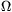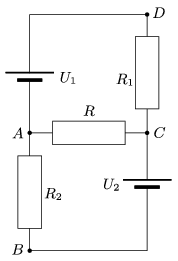Mathematical and Physical Journal
for High Schools
Issued by the MATFUND Foundation
 Already signed up? New to KöMaL?

#Problem P. 4303. (December 2010)

P. 4303. What is the resistance of the resistor R shown in the figure if a) there is no current in the branch AB; b) the current in AC is the greatest; c) the potential difference between points a and C is maximum; d) the power at R is maximum; e) the current in branch DC is maximum; f) the current in AB is the maximum? (Data: U1=60 V, U2=20 V, R1=80, R2=320.)(4 pont)

Deadline expired on January 10, 2011.

Sorry, the solution is available only in Hungarian. Google translation

Megoldás. $\displaystyle a)$ $\displaystyle R=40\ \Omega$

$\displaystyle b)$ $\displaystyle R=0\ \Omega$ (rövidzár)

$\displaystyle c)$ $\displaystyle R=\infty\$ (szakadás)

$\displaystyle d)$ $\displaystyle R=6\ \Omega$

$\displaystyle e)$ $\displaystyle R=0\ \Omega$ (rövidzár)

$\displaystyle f)$ $\displaystyle R=0\ \Omega$ (rövidzár).

### Statistics:

 41 students sent a solution. 4 points: Antalicz Balázs, Ayhan Dániel, Bolgár Dániel, Damokos József, Janzer Barnabás, Laczkó Zoltán Balázs, Máthé László, Nagy Lajos, Park Choong Eun, Pataki Bálint Ármin, Sárvári Péter, Szabó 928 Attila, Szilágyi 585 Dezső, Szilágyi András, Ürge László, Virágh Eszter. 3 points: Broda Balázs, Kozma Bálint, Varga 515 Balázs. 2 points: 4 students. 1 point: 13 students. 0 point: 3 students. Unfair, not evaluated: 2 solutionss.

Problems in Physics of KöMaL, December 2010